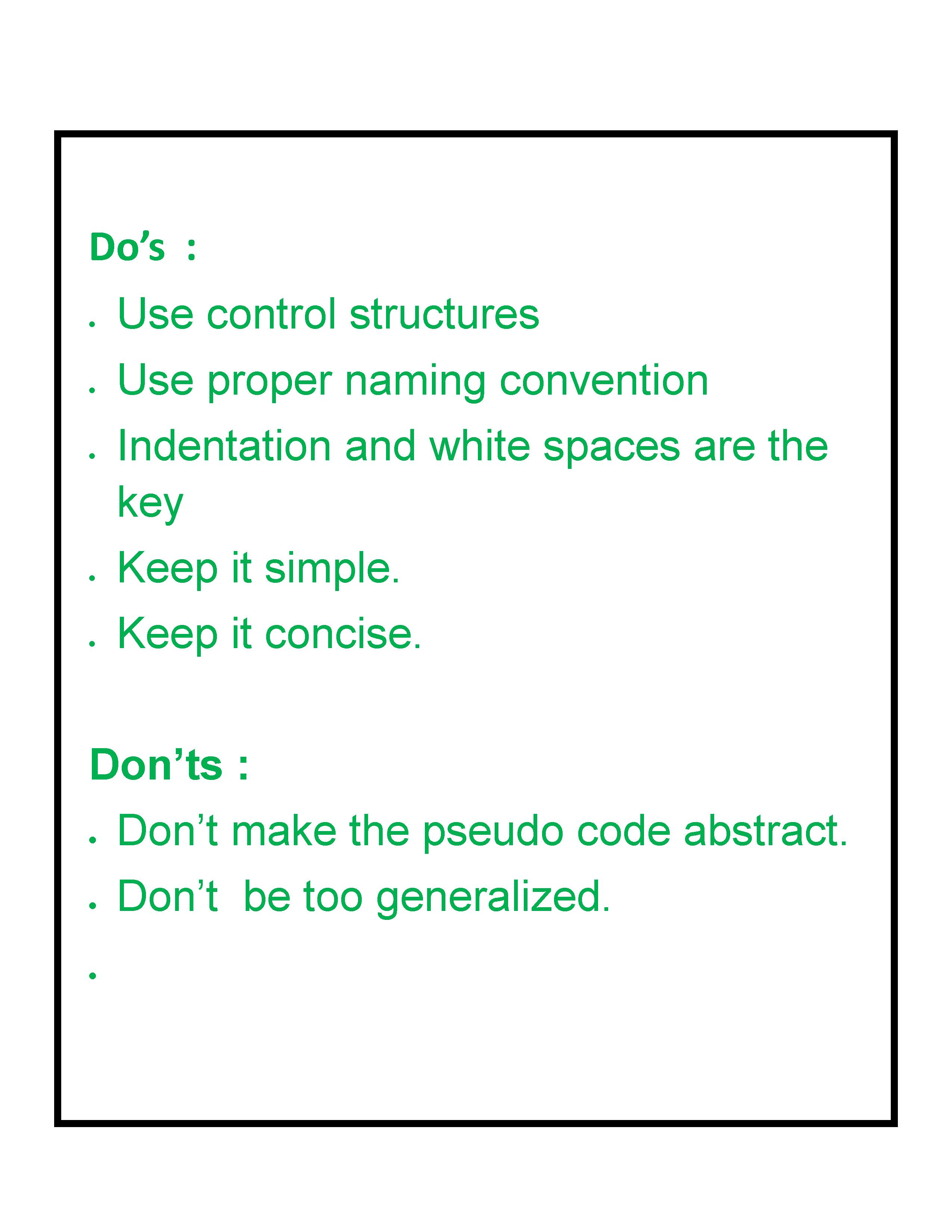Open in App
Not now

# How to write a Pseudo Code?

• Difficulty Level : Easy
• Last Updated : 30 Sep, 2021

Pseudo code is a term which is often used in programming and algorithm based fields. It is a methodology that allows the programmer to represent the implementation of an algorithm. Simply, we can say that it’s the cooked up representation of an algorithm. Often at times, algorithms are represented with the help of pseudo codes as they can be interpreted by programmers no matter what their programming background or knowledge is. Pseudo code, as the name suggests, is a false code or a representation of code which can be understood by even a layman with some school level programming knowledge.

Algorithm: It’s an organized logical sequence of the actions or the approach towards a particular problem. A programmer implements an algorithm to solve a problem. Algorithms are expressed using natural verbal but somewhat technical annotations.

Pseudo code: It’s simply an implementation of an algorithm in the form of annotations and informative text written in plain English. It has no syntax like any of the programming language and thus can’t be compiled or interpreted by the computer.

• Improves the readability of any approach. It’s one of the best approaches to start implementation of an algorithm.
• Acts as a bridge between the program and the algorithm or flowchart. Also works as a rough documentation, so the program of one developer can be understood easily when a pseudo code is written out. In industries, the approach of documentation is essential. And that’s where a pseudo-code proves vital.
• The main goal of a pseudo code is to explain what exactly each line of a program should do, hence making the code construction phase easier for the programmer.

### How to write a Pseudo-code?

1. Arrange the sequence of tasks and write the pseudocode accordingly.
2. Start with the statement of a pseudo code which establishes the main goal or the aim.

Example:

```This program will allow the user to check
the number whether it's even or odd.```
3. The way the if-else, for, while loops are indented in a program, indent the statements likewise, as it helps to comprehend the decision control and execution mechanism. They also improve the readability to a great extent.
```Example:

if "1"
print response
"I am case 1"

if "2"
print response
"I am case 2"
```
4. Use appropriate naming conventions. The human tendency follows the approach to follow what we see. If a programmer goes through a pseudo code, his approach will be the same as per it, so the naming must be simple and distinct.
5. Use appropriate sentence casings, such as CamelCase for methods, upper case for constants and lower case for variables.
6. Elaborate everything which is going to happen in the actual code. Don’t make the pseudo code abstract.
7. Use standard programming structures such as ‘if-then’, ‘for’, ‘while’, ‘cases’ the way we use it in programming.
8. Check whether all the sections of a pseudo code is complete, finite and clear to understand and comprehend.
9. Don’t write the pseudo code in a complete programmatic manner. It is necessary to be simple to understand even for a layman or client, hence don’t incorporate too many technical terms.### Example:

Let’s have a look at this code

## Java

 `// This program calculates the Lowest Common multiple``// for excessively long input values`` ` `import` `java.util.*;`` ` `public` `class` `LowestCommonMultiple {`` ` `    ``private` `static` `long``    ``lcmNaive(``long` `numberOne, ``long` `numberTwo)``    ``{`` ` `        ``long` `lowestCommonMultiple;`` ` `        ``lowestCommonMultiple``            ``= (numberOne * numberTwo)``              ``/ greatestCommonDivisor(numberOne,``                                      ``numberTwo);`` ` `        ``return` `lowestCommonMultiple;``    ``}`` ` `    ``private` `static` `long``    ``greatestCommonDivisor(``long` `numberOne, ``long` `numberTwo)``    ``{`` ` `        ``if` `(numberTwo == ``0``)``            ``return` `numberOne;`` ` `        ``return` `greatestCommonDivisor(numberTwo,``                                     ``numberOne % numberTwo);``    ``}``    ``public` `static` `void` `main(String args[])``    ``{`` ` `        ``Scanner scanner = ``new` `Scanner(System.in);``        ``System.out.println(``"Enter the inputs"``);``        ``long` `numberOne = scanner.nextInt();``        ``long` `numberTwo = scanner.nextInt();`` ` `        ``System.out.println(lcmNaive(numberOne, numberTwo));``    ``}``}`

And here’s the Pseudo Code for the same.

 `This program calculates the Lowest Common multiple ``for excessively long input values`` ` `function lcmNaive(Argument one, Argument two){`` ` `    ``Calculate the lowest common variable of Argument``    ``1 and Argument 2 by dividing their product by their``    ``Greatest common divisor product`` ` `    ``return lowest common multiple``end``}``function greatestCommonDivisor(Argument one, Argument two){``    ``if Argument two is equal to zero``        ``then return Argument one`` ` `    ``return the greatest common divisor`` ` `end``}`` ` `{``In the main function``    ` `   ``print prompt "Input two numbers"``        ` `   ``Take the first number from the user``   ``Take the second number from the user`` ` `   ``Send the first number and second number ``   ``to the lcmNaive function and print``   ``the result to the user   ``}`

My Personal Notes arrow_drop_up